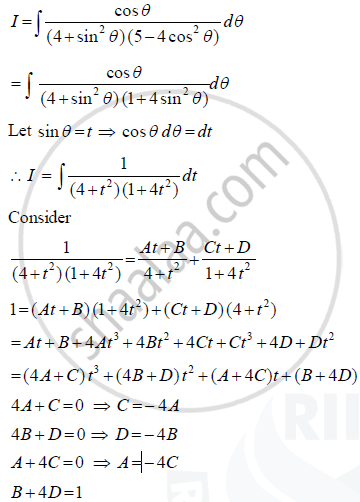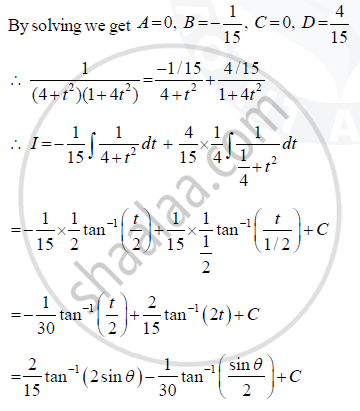Share

# Find Integral Cos Theta by 4 + Sin Square Thetax5 - 4 Cos Square Theta - CBSE (Commerce) Class 12 - Mathematics

ConceptProperties of Indefinite Integral

#### Question

Find int (cos theta)/((4 + sin^2 theta)(5 - 4 cos^2 theta)) d theta

#### SolutionIs there an error in this question or solution?

#### Video TutorialsVIEW ALL 

Solution Find Integral Cos Theta by 4 + Sin Square Thetax5 - 4 Cos Square Theta Concept: Properties of Indefinite Integral.
S